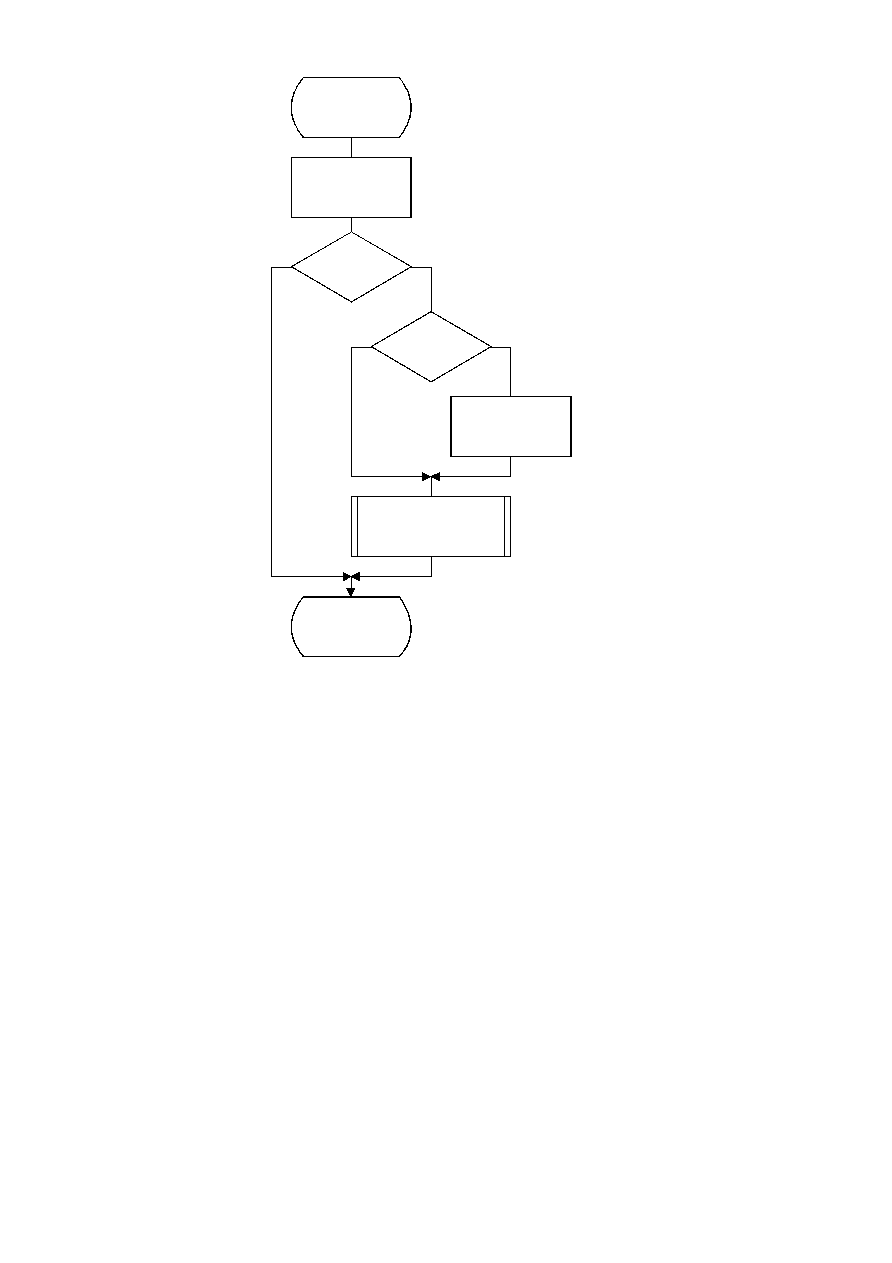ISO/IEC 10918-1 : 1993(E)
TISO1050-93/d042
Code_MPS(S)
A = A ­ Qe(S)
No
No
Yes
Yes
A < X'8000'
?
A < Qe(S)
?
C = C + A
A = Qe(S)
Estimate_Qe(S)_after_MPS
Renorm_e
Done
Figure D.4 ­ Code_MPS(S) procedure with conditional MPS/LPS exchange
Figure D.4 [D42], = 16.5 cm = 645.%
D.1.5
Probability estimation in the encoder
D.1.5.1
Probability estimation state machine
The probability estimation state machine consists of a number of sequences of probability estimates. These sequences are
interlinked in a manner which provides probability estimates based on approximate symbol counts derived from the
arithmetic coder renormalization. Some of these sequences are used during the initial "learning" stages of probability
estimation; the rest are used for "steady state" estimation.
Each entry in the probability estimation state machine is assigned an index, and each index has associated with it a
Qe value and two Next_Index values. The Next_Index_MPS gives the index to the new probability estimate after an MPS
renormalization; the Next_Index_LPS gives the index to the new probability estimate after an LPS renormalization. Note
that both the index to the estimation state machine and the sense of the MPS are kept for each context-index S. The sense
of the MPS is changed whenever the entry in the Switch_MPS is one.
The probability estimation state machine is given in Table D.3. Initialization of the arithmetic coder is always with
an MPS sense of zero and a Qe index of zero in Table D.3.
The Qe values listed in Table D.3 are expressed as hexadecimal integers. To approximately convert the 15-bit integer
representation of Qe to a decimal probability, divide the Qe values by (4/3)
×
(X'8000').
CCITT Rec. T.81 (1992 E)
59